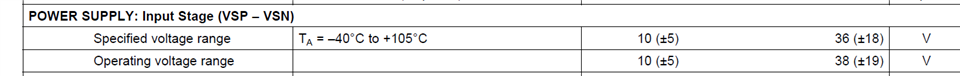If you have a related question, please click the "Ask a related question" button in the top right corner. The newly created question will be automatically linked to this question.

# PGA281: what is the voltage range of VSP and VSN? do I must use a negative power to VSN?

Part Number: PGA281

what is the voltage range of VSP and VSN? i checked the below datasheet, but still confused. is that means VSP is 10V to 36V more than VSN is OK?   can I use VSP=10V and VSN=0V ? ( single power)  or I should use VSP=5V, VSN=-5V( positive and negative power)?• and what is the total operating current of PGA281?  I mean in working mode, not in quiescent mode

• HI YY,

The PGA281 can be powered with unipolar supply, positive supply where VSN +0V and VSP=+10V; also, the device can be powered with bipolar or positive/negative +/-5V supplies as shown in the datasheet specification above.

Also, please keep in mind the input voltage range of the instrumentation amplifier: both INN and INP inputs must remain at least 2.5V from the input stage supply rails for the device to remain in the linear region and operate properly.  In other words, the allowed voltage input range of the INN and INP inputs is:

(VSN) + 2.5V <  Input voltage Range < (VSP) - 2.5V

For example, if your application requires to measure input voltages referred to GND where one of the inputs is connected to ground (INN=GND), then the device needs to be powered with bipolar VSP/VSN positive/negative supplies so the inputs remain inside the allowed input voltage range.

The quiescent current is the current consumption of the PGA281 when the device is turned on and the output is unloaded.  If you load the amplifier, load current is added to the total current consumption. As shown in the datasheet, the PGA281 in quiescent current consumption of the device is a typical 2.4mA and 2.1mA at the input stage supplies (VSP/VSN) and 750uA at the output stage supplies (unloaded). If the PGA281 output stage is loaded, for example, if the load at the VSOP output is 1mA, then this additional output load current is added the total current consumption of the circuit.

Thank you and Regards,

Luis

• thanks. so in my design, I will use VSP=5V, VSN=-5V, VSOP=5V, VSON=0V, DVDD=3.3V, is that Ok?

• HI YY,

Yes, that will work.

In this case, the allowed input voltage range of the instrumentation amplifier inputs INN/INP is +/-2.5V  (or 2.5V away from the VSP/VSN input stage supplies).

Also, please ensure the VOCM pin is set to the required voltage.

If the output stage supplies are set to, VSOP=+5V and VSON=0V, the VOCM needs to be driven to a voltage that is typically close to mid output stage supply. The PGA281 output produces a symmetrical fully-differential signal centered around the VOCM voltage, in other words, the PGA281 output common-mode voltage level is controlled by the VOCM pin.

Thank you and Regards,

Luis

• yes. i use two 100K ohm resistor divider from 5V to get 2.5V for VOCM.

• Hi YY,

Sounds good, feel free to post if you have further questions.

Kind Regards,

Luis